# Cyclotron Basic Construction and Working Principle

Before understanding the basic working principle of Cyclotron it is necessary to understand force on a moving charged particle in a magnetic field and also motion of the charged particle in the magnetic field.

### Force on a Moving Charged Particle in a Magnetic Field

When a current carrying conductor of length L metre with current I ampere placed perpendicularly in a magnetic field of flux density B Weber per metre square, then the force rather to say magnetic force acting on the conductor would be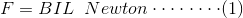Now, let us consider there are total N number of mobile free electrons in the conductor lie in length L metre causing the current I ampere.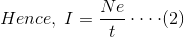Where, e is the electric charge of one electron and it is 1.6 × 10-19 coulomb.
Now from equation (1) and (2) we get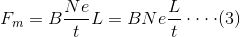Here, N number of electrons causing current I ampere, and consider that they travel length L metre in time t, therefore drift velocity of the electrons would be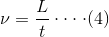From equation (3) and (4), we get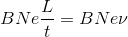It is the force acting on N number of electrons in the magnetic field hence force on a single electron in that magnetic field can be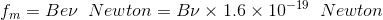### Motion of Charged Particle in a Magnetic Field

When a charged particle moves in a magnetic field, there would be extreme two conditions. The particle moves either along the direction of the magnetic field or it moves perpendicular to the magnetic field.
When the particle moves along the axis of the direction of magnetic field, magnetic force acting on it,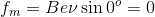Hence there will be no force acting on the particle, hence no change in the velocity of particle and hence it moves in straight line with constant speed.

Now if the charged particle moves perpendicular to the magnetic field then there will be no change in the speed of the particle. This is because the force acting on the particle is perpendicular to the motion of the particle hence the force will not do any work on the particle so there will be no change in the speed of the particle.
But this force acting on the particle perpendicular to its motion and the direction of the motion of the particle will change continuously. As a result the particle will move in a in the field in a circular path of a constant radius with constant speed.
If the radius of the circular motion is R metre then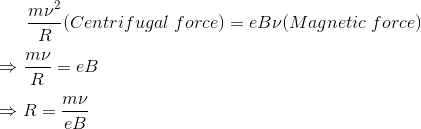Now,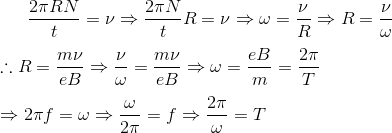Hence radius of the motion depends upon the velocity of the motion.
Angular speed and time period are constant.

## Basics Principle of Cyclotron

This concept of motion of charged particle in a magnetic field was successfully employed in an apparatus called cyclotron. Conceptually this device is very simple but it has huge uses in the field of engineering, physics and medicine. This is a charged particle accelerating device. The motion of the charged particle under perpendicular magnetic field is solely applied in the apparatus named cyclotron.

### Construction of Cyclotron

This device basically has three main constructional parts

1. Large sized electromagnet to create uniform magnetic field in between its two face-to-face placed magnetic opposite poles.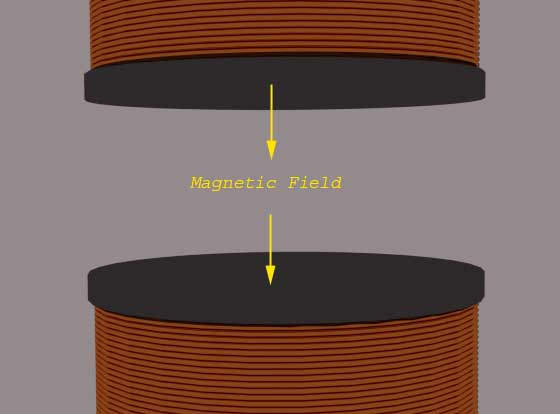2. Two low height hollow half cylinders made of high conductive metals. These components of cyclotron are called Dees.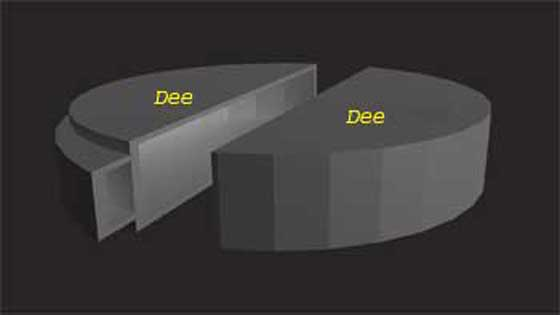3. A high-frequency alternating high voltage source.

#### Constructional Details

The Dees are placed face to face in between the electromagnetic poles. The dees are so placed, that the straight edge will be face-to-face with small gap between them. Also the magnetic flux of the electromagnet cut these Dees exact perpendicularly. Now these two Dees are connected to two terminals of an alternating voltage source so that if one Dees is in the positive potential than other will be in exact opposite negative potential at same time. As the source is alternating the potential of the Dees are altered according to the frequency of the source. Now if a charged particle is thrown from a point near to the centre of one Dees with certain velocity V1. As the movement of the particle now perpendicular to the externally applied magnetic field, there will be no change of velocity but the charged particle will follow a circular path of radius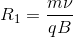Where, m gram is the mass and q coulomb is the charge of the thrown particle and B Weber/metre2 is the flux density of externally applied perpendicular magnetic field.
After travelling π radians or 180o with radius R1 the charged particle comes to the edge of the Dee. Now the time period and frequency of the applied voltage source is so adjusted with the time period of circular motion that is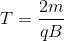That the polarity of the other Dee becomes opposite that of the charged of the particle. Hence due to attraction of the Dee ahead the moving particle and also due to repulsion of the Dee in which the particle is now situated, the particle gets extra kinetic energy.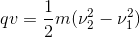Where ν1 is the velocity of the particle at previous Dee and ν2 is the velocity of the particle in next Dee. Now the particle will move with this greater velocity with radius R2 metre.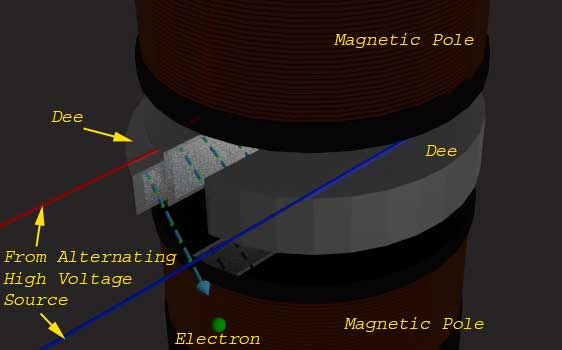Again due to constant perpendicular magnetic field the particle travels another half cycle with this new radius R2 metre and comes to the edge of present Dee. When it comes to this edge, the ahead Dee again becomes in opposite polarity of the behind and the particle crosses the gap between Dees with gain of kinetic energy qV and hence again there is gain of velocity and radius of the circularly travelling charged particle. In this way the charged particle follows a spiral path of motion with continually increasing velocity. Therefore the charged particle gets sufficiently high required velocity before leaving the cyclotron gun head.
The frequency of voltage source say f.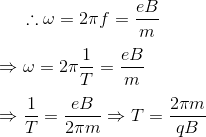Here, 2π is constant, m, q and B are known hence T can be calculated and hence frequency of the voltage source would be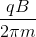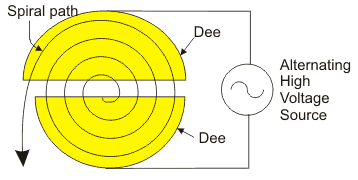## Application of Cyclotron

There are mainly two types of application of cyclotron. One is in lavatory of different physics experiments when highly accelerated photons are required. Also highly accelerated photons are used to irradiate tissues.

Want To Learn Faster? 🎓
Get electrical articles delivered to your inbox every week.
No credit card required—it’s 100% free.